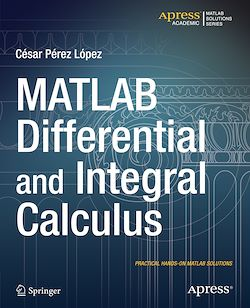# MATLAB Differential and Integral Calculus

-English
228 Pages

Description

MATLAB is a high-level language and environment for numerical computation, visualization, and programming. Using MATLAB, you can analyze data, develop algorithms, and create models and applications. The language, tools, and built-in math functions enable you to explore multiple approaches and reach a solution faster than with spreadsheets or traditional programming languages, such as C/C++ or Java.

MATLAB Differential and Integral Calculus introduces you to the MATLAB language with practical hands-on instructions and results, allowing you to quickly achieve your goals. In addition to giving a short introduction to the MATLAB environment and MATLAB programming, this book provides all the material needed to work with ease in differential and integral calculus in one and several variables. Among other core topics of calculus, you will use MATLAB to investigate convergence, find limits of sequences and series and, for the purpose of exploring continuity, limits of functions. Various kinds of local approximations of functions are introduced, including Taylor and Laurent series. Symbolic and numerical techniques of differentiation and integration are covered with numerous examples, including applications to finding maxima and minima, areas, arc lengths, surface areas and volumes. You will also see how MATLAB can be used to solve problems in vector calculus and how to solve differential and difference equations.

Subjects

##### IT systems

Informations